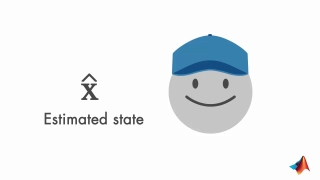# Understanding Kalman Filters, Part 2: State Observers8 min
Beginner
Video
Theory

Learn the working principles of state observers, and discover the math behind them. State observers are used for estimating the internal states of a system when you can’t directly measure them.

You will learn how a state observer uses the input and output measurements to estimate system states. The example will walk you through the mathematical derivation of a state observer.

You will discover how the state observer utilizes feedback control to drive the estimated states to the true states. Kalman filtering provides an optimal way of choosing the gain of this feedback controller.

This resource is included in the following topics and journeys: